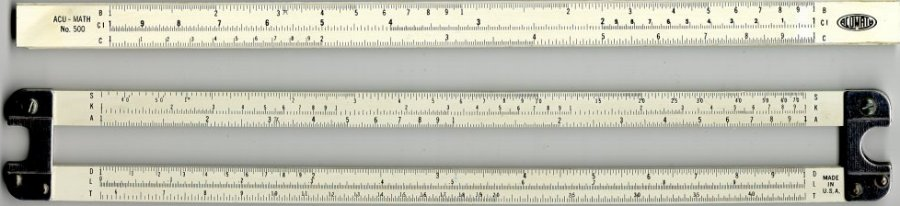# Logarithmic Properties

## The Definition of a Logarithm

 A logarithm is an exponent.

Note, the above is not a definition, merely a pithy description.

Just as subtraction is the inverse operation of addition, and taking a square root is the inverse operation of squaring, exponentiation and logarithms are inverse operations. Finding an antilog is the inverse operation of finding a log, so is another name for exponentiation. However, historically, this was done as a table lookup. Some history was given earlier and the formal definition is repeated below, this time with restrictions.

 y = logbx if and only if by = x, where x > 0, b > 0, and b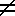1.

As noted above, the base can be any positive number (except 1). However, two choices are most usual: 10 and e=2.718281828.... Logs to the base 10 are often call common logs, whereas logs to the base e are often call natural logs. Logs to the bases of 10 and e are now both fairly standard on most calculators. Often when taking a log, the base is arbitrary and does not need to be specified. However, at other times it is necessary and must be assumed or specified.

 At the high school level only, log x consistantly means log10x. In college, especially in mathematics and physics, log x consistantly means logex. A popular notation (despised by some) is: ln x means logex.

To calculate logs to other bases, the change of base rule below (#4) should be used. It is only multiplication by a constant (1 / logab).

## The Four Basic Properties of Logs

 logb(xy) = logbx + logby. logb(x/y) = logbx - logby. logb(xn) = n logbx.           logbx = logax / logab.

These four basic properties all follow directly from the fact that logs are exponents. In words, the first three can be remembered as: The log of a product is equal to the sum of the logs of the factors. The log of a quotient is equal to the difference between the logs of the numerator and demoninator. The log of a power is equal to the power times the log of the base.

Additional properties, some obvious, some not so obvious are listed below for reference. Number 6 is called the reciprocal property.

 logb1 = 0.           logbb = 1.           logbb2 = 2.          logbbx = x.          blogbx = x.           logab = 1/logba.

## The Slide Rule

The invention of logs was followed quickly by the invention of the slide rule. Slide rules simplify multiplication and division by converting these operations into addition and subtraction. This is done by placing the numbers on a scale which is logarithmic. Given below are the logs of some small integers.
n log10n logen 1 0.000 0.000 2 0.301 0.693 3 0.477 1.099 4 0.602 1.386 5 0.699 1.609 6 0.778 1.792 7 0.845 1.946 8 0.903 2.079 9 0.954 2.197 10 1.000 2.303

From this we can readily verify such properties as: log 10 = log 2 + log 5 and log 4 = 2 log 2. These are true for either base. In fact, the useful result of 103 = 1000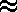1024 = 210 can be readily seen as 10 log10 23.

The slide rule below is presented in a disassembled state to facilitate cutting. (Also, by putting it below, it will be at the bottom of page 3 and have blank paper behind it.) The portion above slides in the center of the portion below and should be printed, then cut out for demonstration purposes as follows.

1. Align the left 1 on the D scale with the 2 on the C scale. Observe the number above 4 of the D scale on the C scale. Since these numbers are laid out on a logorithmic scale, you have shown that log 2 + log 4 = log (2×4) = log 8. Circle that 8.

2. Align the right 1 on the D scale with the 4 on the C scale. Observe the number below the left 1 on the C scale. You have just shown that log 10 - log 4 = log 2.5. Circle that 2.5.

3. Align the D scale and A scale. The A scale is laid out similarily, except there are two cycles present. Observe the number just above the 9 on the D scale. You have just shown that 2 log 9 = log 92 = log 81. Circle that 81.

4. See how the K scale can be used to cube things.

5. Notice how the CI scale can also be used to divide.

Normally there is a cursor (the original meaning, not the kind blinking on the computer screen) present which allows one to get about three decimal places of accuracy, hence the term slide rule accuracy.

## Applications of Logarithms

Logs are used in a variety of applications in sciences, some of the most common are: measuring loudness (decibels), measureing earthquake intensity (Richter scale), radioactive decay, and acidity (pH= -log10[H+]). They are essential in mathematics to solve certain exponential-type problems.

Following, is an interesting problem which ties the quadratic formula, logarithms, and exponents together very neatly.

log(2x+2) + log x - log(12) = 0
Simplify the logarithms by combining them.
log(2x2 + 2x) - log(12) = 0
log((2x2 + 2x)/12) = 0

After dividing by 2, exponentiate both sides (the base b is arbitrary, since it was not specified above)!
(x2 + x)/6 = b0
(x2 + x)/6 = 1
x2 + x = 6
x2 + x - 6 = 0
(x + 3)(x - 2) = 0
x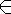{-3, 2}

Blank space so when printed with Mozilla (oops, no boxes) it is in back of the slide rule.

However x-3 since the domain of log is only the positive reals. (bx can never be a negative number with b > 0).

The next example (6.11#51) combines logarithms with simultaneous equations. It is also very convenient to introduce the concept of substitution, which is so useful in calculus.

log9x + logy8 = 2.
logx9 + log8y = 8/3.

Let u=log9x and v=log8y. By the reciprocal property above, 1/u=logx9 and 1/v=logy8.

We can rewrite our equations now as:

u + 1/v = 2
1/u + v = 8/3

Solving by substitution, u = 2 - 1/v, thus:
1/(2 - 1/v) + v = 8/3.
3(1 + 2v - 1) = 8(2 - 1/v)
6v2 = 16v - 8.
6v2 - 16v + 8 = 0.
3v2 - 8v + 4 = 0.
To this we apply the quadratic formula and find that
v = (8 ±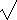(64 - 48))/6.
= (8 ± 4)/6 or 2, 2/3.
Thus u = 3/2 or 1/2       or (u, v)={(3/2, 2), (1/2, 2/3)}
Thus (x, y) = {(27, 64), (3, 4)}

BACK HOMEWORK ACTIVITY CONTINUE